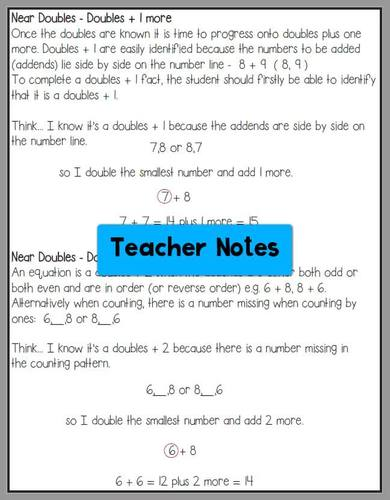# Addition Strategies for Number Fact Fluency Distance LearningSubject
Resource Type
File Type

Zip

(74 MB|114 pages)
Standards
Also included in:
1. Knowing and applying addition strategies and subtraction strategies help improve fact fluency. We all want our young learners to confidently answer their number facts with no hesitating or counting on fingers. To be able to fluently add or subtract within twenty using mental math strategies and know
\$15.00
\$12.50
Save \$2.50
2. Knowing and applying addition strategies and subtraction strategies help improve fact fluency. We all want our young learners to confidently answer their number facts with no hesitating or counting on fingers. To be able to fluently add or subtract within twenty using mental math strategies and know
\$31.00
\$22.00
Save \$9.00
• Product Description
• Standards

Addition fact fluency – we all want our young learners to confidently answer their addition facts with no hesitating or counting on fingers. To be able to fluently add within twenty using mental math strategies and know from memory all sums of two one-digit numbers is math standard for all second graders.

You can help them become fluent and increase their confidence in math by teaching them addition mental math strategies.

Distance Learning

This pack of 60 single player or partner games and activities is perfect for teachers organizing distance learning or parents looking for at home learning activities.

(⭐ Looking for addition strategy games specifically for Google Classroom? Try Addition Strategies Distance Learning pack 1 or Addition Strategies Distance Learning pack 2)

This math unit contains:

⇒ Teacher explanation for each strategy. (Great for parents too!)

⇒ A flip book - use as a reference tool for when the class is learning each addition strategy.

⇒ 5 activities (a total of 60 activities) which can be used independently or in pairs for consolidating each of the following strategies:

- Making 10

- Counting on 1

- Counting on 2

- Counting on 3

- Counting on 1, 2 and 3

- Doubles

- Near doubles (doubles + 1)

- Near doubles ( doubles + 2)

⇒ 5 activities (a total of 25 activities) which can be used independently or in pairs to consolidate the strategies when ...

⇒ Interactive workbook pockets for using the above strategies. Students answer the number facts and sort them into the strategy pockets.

(British English is included.)

It's the perfect companion to Subtraction Strategies for Number Fact Fluency

For more practice with addition strategies try:

Addition Strategies - Games and Posters

Addition Strategies - Homework Games 2

Addition facts to 20 - Bump games

_______________________________________________________________________________

Become a follower and take advantage of my Early Bird Specials...all new products, excluding bundles, will be 50% off for the first 24 hours after being listed.

Fluently add and subtract within 20 using mental strategies. By end of Grade 2, know from memory all sums of two one-digit numbers.
Add and subtract within 20, demonstrating fluency for addition and subtraction within 10. Use strategies such as counting on; making ten (e.g., 8 + 6 = 8 + 2 + 4 = 10 + 4 = 14); decomposing a number leading to a ten (e.g., 13 - 4 = 13 - 3 - 1 = 10 - 1 = 9); using the relationship between addition and subtraction (e.g., knowing that 8 + 4 = 12, one knows 12 - 8 = 4); and creating equivalent but easier or known sums (e.g., adding 6 + 7 by creating the known equivalent 6 + 6 + 1 = 12 + 1 = 13).
Relate counting to addition and subtraction (e.g., by counting on 2 to add 2).
Understand subtraction as an unknown-addend problem. For example, subtract 10 – 8 by finding the number that makes 10 when added to 8.
Apply properties of operations as strategies to add and subtract. If 8 + 3 = 11 is known, then 3 + 8 = 11 is also known. (Commutative property of addition.) To add 2 + 6 + 4, the second two numbers can be added to make a ten, so 2 + 6 + 4 = 2 + 10 = 12. (Associative property of addition.)
Total Pages
114 pages
N/A
Teaching Duration
N/A
Report this Resource to TpT
Reported resources will be reviewed by our team. Report this resource to let us know if this resource violates TpT’s content guidelines.